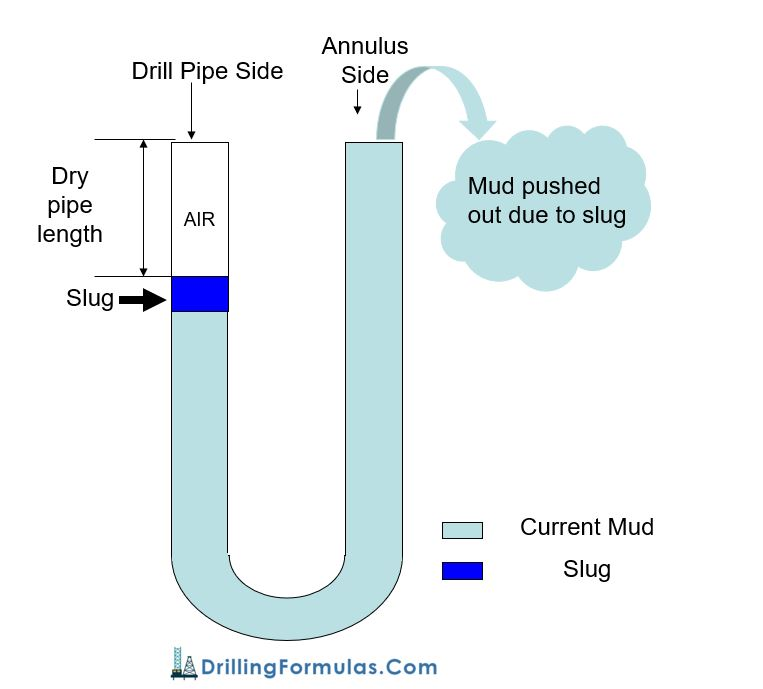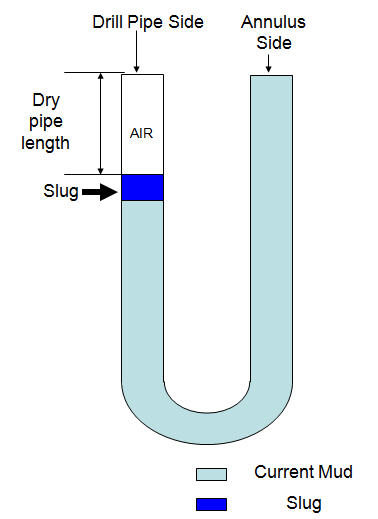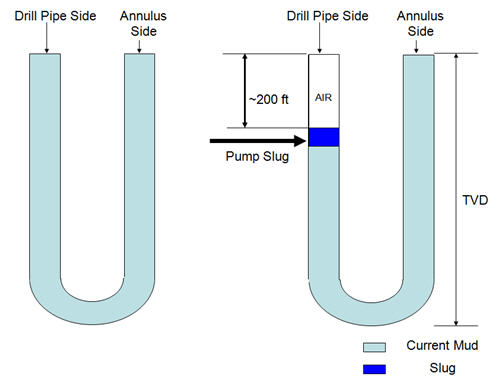## Volume Gain from SlugSlug mud is typically pumped into the drill string in order to push the mud inside the drill pipe down so the drill pipe will be clean and ready for pulling out of hole. Since slug is heavier, it will push the lighter mud out of the well. Figure 1 demonstrates how slug displaces current mud out of hole.Figure 1 – Diagram Shows Volume Gain from Slug

## Weight of slug required for desired length of dry pipe with set volume of slugYou can determine how much slug weight required in order to achieve desired length of dry pipe with certain slug volume that you will use.## Oilfield Unit

Step 1 Determine Length of slug in drill pipe in ft:

Length of slug in drill pipe in ft = slug volume in bbl ÷ drill pipe capacity in bbl/ft

Step 2 Determine hydrostatic pressure required to give desired dry pipe drill pipe:

Hydrostatic Pressure in psi = mud weight in ppg × 0.052 × desired length of dry pipe

Step 3 Determine slug weight needed in ppg:

Slug weight in ppg = (Hydrostatic Prำssure (from step 2) ÷ 0.052 ÷ Length of slug in ft (step1)) + mud weight, ppg, in hole

Example: Determine slug weight required for the following data:

Desired length of dry pipe = 200 ft

Mud weight in hole = 12.0 ppg

Drill pipe capacity = 0.016 bbl/ft

Volume of slug = 20 bbl

Step 1 – Determine Length of slug inside drill pipe in ft:

Slug length = 20 bbl ÷ 0.016 bbl/ft

Slug length = 1250 ft

Step 2 – Determine hydrostatic pressure required to give desired dry pipe drill pipe

Hydrostatic Pressure in psi = 12.0 × 0.052 × 200

Hydrostatic Pressure in psi = 124.8 psi

Step 3 – Determine slug weight needed in ppg:

Slug weight in ppg = (124.8 ÷ 0.052 ÷ 1250) + 12.0

Slug weight in ppg = 13.9 ppg

## Metric Unit

Step 1 Determine Length of slug in drill pipe in meter (m):

Length of slug in drill pipe in m = slug volume in m³ ÷ drill pipe capacity in m³/m

Step 2 Determine hydrostatic pressure required to give desired dry pipe drill pipe:

Hydrostatic Pressure in psi = mud weight in kg/m³ × 0.00981 × desired length of dry pipe in m

Step 3 Determine slug weight needed in ppg:

Slug weight in ppg = (Hydrostatic Pressure (from step 2) ÷ 0.00981÷ Length of slug in m (step1)) + mud weight, kg/m³, in hole

Example: Determine slug weight required for the following data:

Desired length of dry pipe = 120 m

Mud weight in hole = 1,400 kg/m³

Drill pipe capacity = 0.007824 m³/m

Volume of slug = 4.5 m³

Step 1 – Determine Length of slug inside drill pipe in ft:

Slug length = 4.5 m³÷ 0.007824 m³/m

Slug length = 575 m

Step 2 – Determine hydrostatic pressure required to give desired dry pipe drill pipe

Hydrostatic Pressure in psi = 1,400 × 0.00981 × 120

Hydrostatic Pressure in psi = 1,648 KPa

Step 3 – Determine slug weight needed in ppg:

Slug weight in ppg = (1,648 ÷ 0.00981 ÷ 575) + 1,400

Slug weight in ppg = 1692 kg/m³Please find the excel sheet used to calculate Weight of slug required for a desired length of dry pipe with a set volume of slug.Ref books:

Lapeyrouse, N.J., 2002. Formulas and calculations for drilling, production and workover, Boston: Gulf Professional publishing.

Bourgoyne, A.J.T., Chenevert , M.E. & Millheim, K.K., 1986. SPE Textbook Series, Volume 2: Applied Drilling Engineering, Society of Petroleum Engineers.

Mitchell, R.F., Miska, S. & Aadny, B.S., 2011. Fundamentals of drilling engineering, Richardson, TX: Society of Petroleum Engineers.

## Barrels of slug required for desired length of dry pipeWhat is slug? Slug: It is heavy mud which is used to push lighter mud weight down before pulling drill pipe out of hole. Slug is used when pipe became wet while pulling out of hole. This article will demonstrate you how to calculate how many barrel of volume slug required for desired light of dry pipe.

Normally, 1.5 to 2 PPG over current mud weight is a rule of thumb to decide how much weight of slug should be. For example, current mud weight is 10 PPG. Slug weight should be about 11.5 to 12 PPG.  Generally, slug is pumped to push mud down approximate 200 ft and slug volume can be calculated by applying a concept of U-tube (See Figure below).

Volume of slug required for required length of dry pipe can be calculated by this following equations:

## Oilfield Unit

Step 1: Determine hydrostatic pressure required to give desired drop inside drill pipe:

Hydrostatic Pressure in psi = mud weight in ppg × 0.052 × ft of dry pipe

Step 2: Determine difference in pressure gradient between slug weight and mud weight:

Pressure gradient difference in psi/ft = (slug weight in ppg – mud weight in ppg) × 0.052

Step 3: Determine length of slug in drill pipe:

Slug length in ft = Hydrostatic Pressure in psi (in step 1) ÷ Pressure gradient difference in psi/ft (step 2)

Step 4: Slug volume required in barrels:

Slug volume in barrel = Slug length in ft × drill pipe capacity in bbl/ft

Example: Determine the barrels of slug required for the following:

Desired length of dry pipe = 200 ft

Drill pipe capacity = 0.016 bbl/ft

Mud weight = 10.0 ppg

Slug weight = 11.5 ppgStep 1 Hydrostatic pressure required:

Hydrostatic Pressure in psi = 10.0 ppg × 0.052 × 200 ft

Hydrostatic Pressure in psi = 104 psi

Step 2 differences in pressure gradient between slug weight and mud weight:

Pressure gradient difference in psi/ft = (11.5 ppg – 10.0 ppg) × 0.052

Pressure gradient difference in psi/ft = 0.078 psi/ft

Step 3 length of slug in drill pipe:

Slug length in ft = 104 psi ÷ 0.078 psi/ft

Slug length in ft = 1,333 ft

Step 4 Slug volume required in barrels:

Slug volume required = 1,333 ft × 0.016 bbl/ft

Slug volume required = 21.3 bbl

## Metric Unit

Step 1: Determine hydrostatic pressure required to give desired drop inside drill pipe:

Hydrostatic Pressure in kPa = mud weight in kg/m³ × 0.00981 × length of dry pipe in m

Step 2: Determine difference in pressure gradient between slug weight and mud weight:

Pressure gradient difference in kPa/m = (slug weight in kg/m³ – mud weight in kg/m³) × 0.00981

Step 3: Determine length of slug in drill pipe:

Slug length in m = Hydrostatic Pressure in kPa (in step 1) ÷ Pressure gradient difference in kPa/m(step 2)

Step 4: Slug volume required in barrels:

Slug volume in m³ = Slug length in m × drill pipe capacity in m³/m

Example: Determine the barrels of slug required for the following:

Desired length of dry pipe = 120 m

Drill pipe capacity = 0.00782 m³/m

Mud weight = 1,380 kg/m³

Slug weight = 1,500 kg/m³

Step 1 Hydrostatic pressure required:

Hydrostatic Pressure in psi = 1,380 kg/m³ × 0.00981 × 120 m

Hydrostatic Pressure in psi = 1,625 kPa

Step 2 differences in pressure gradient between slug weight and mud weight:

Pressure gradient difference in kPa/m = (1,500 kg/m³ – 1,380 kg/m³ ) × 0.00981

Pressure gradient difference in kPa/m = 1.1772 kPa/m

Step 3 length of slug in drill pipe:

Slug length in ft = 1,625 kPa ÷ 1.1772 kPa/m

Slug length in ft = 1,380 m

Step 4 Slug volume required in barrels:

Slug volume required = 1,380 m × 0.00782 m³/m

Slug volume required = 10.79 m³

Ref books:

Lapeyrouse, N.J., 2002. Formulas and calculations for drilling, production and workover, Boston: Gulf Professional publishing.

Bourgoyne, A.J.T., Chenevert , M.E. & Millheim, K.K., 1986. SPE Textbook Series, Volume 2: Applied Drilling Engineering, Society of Petroleum Engineers.

Mitchell, R.F., Miska, S. & Aadny, B.S., 2011. Fundamentals of drilling engineering, Richardson, TX: Society of Petroleum Engineers.